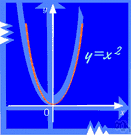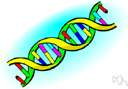# mapping

Also found in: Thesaurus, Medical, Encyclopedia.

## map·ping

(măp′ĭng)
n.
1. The act or process of making a map.
2. Mathematics See function.

## mapping

(ˈmæpɪŋ)
n
(Mathematics) maths another name for function4

## map•ping

(ˈmæp ɪŋ)

n.
1. the act or operation of making maps.
[1765–75]
ThesaurusAntonymsRelated WordsSynonymsLegend:
 Noun 1mapping - (mathematics) a mathematical relation such that each element of a given set (the domain of the function) is associated with an element of another set (the range of the function)multinomial, polynomial - a mathematical function that is the sum of a number of termsmath, mathematics, maths - a science (or group of related sciences) dealing with the logic of quantity and shape and arrangementmathematical relation - a relation between mathematical expressions (such as equality or inequality)expansion - a function expressed as a sum or product of terms; "the expansion of (a+b)^2 is a^2 + 2ab + b^2"inverse function - a function obtained by expressing the dependent variable of one function as the independent variable of another; f and g are inverse functions if f(x)=y and g(y)=xKronecker delta - a function of two variables i and j that equals 1 when i=j and equals 0 otherwisemetric, metric function - a function of a topological space that gives, for any two points in the space, a value equal to the distance between themtransformation - (mathematics) a function that changes the position or direction of the axes of a coordinate systemisometry - a one-to-one mapping of one metric space into another metric space that preserves the distances between each pair of points; "the isometries of the cube"operator - (mathematics) a symbol or function representing a mathematical operationcircular function, trigonometric function - function of an angle expressed as a ratio of the length of the sides of right-angled triangle containing the anglethreshold function - a function that takes the value 1 if a specified function of the arguments exceeds a given threshold and 0 otherwiseexponential, exponential function - a function in which an independent variable appears as an exponent 2mapping - (genetics) the process of locating genes on a chromosomechromosome mappingprocedure, process - a particular course of action intended to achieve a result; "the procedure of obtaining a driver's license"; "it was a process of trial and error"genetic science, genetics - the branch of biology that studies heredity and variation in organisms
Translations
kartoituskuvaus
preslikavanje

## mapping

n (Comput) → Abbildung f, → Mapping nt; (Math, Comput) → Zuordnung f

## mapping

[ˈmæpɪŋ] n (Math) →
References in periodicals archive ?
In addition to toxicity screening, the unique genetic structure of the DO mice can be used to perform high-resolution linkage mapping to identify genetic variants that determine individual responses to toxicant exposures.
It is this polymorphic nature that makes these markers ideal for linkage mapping because their multiallelic status allows for a high information content, facilitating the detection of segregating alleles (Hauser & Seeb 2008).
Most of these chapters discuss statistical and computational methods for linkage mapping and association studies in experimental species and humans.
Secondly, map length may be inflated by the linkage mapping program MAPMAKER (Sybenga, 1996).
The search for alcoholism susceptibility genes centers on two major techniques, linkage mapping and the candidate gene approach.
The instrument can sequence plasmid, BAC end, and double-stranded templates and can be used with PE Biosystems' BigDye Primer and Terminator chemistries and Linkage Mapping Set Version 2 fragment analysis chemistries as well as with various other chemistries.
The invention of linkage mapping in 1912 - largely the inspiration of graduate students Alfred Sturtevant and Calvin Bridges, according to Kohler - rapidly obliged the Drosophilists to "standardize" the fly, that is to remove all unwanted genetic variation and to create an instrument for the recombinant mapping of the genome.
The developed SSR markers will be helpful for further studies on population genetics, genetic linkage construction and chromosome linkage mapping in the species.
The flow map of the SNP marker selection process for linkage mapping is illustrated in Figure 1.
The objectives of this study are to (1) isolate and characterize microsatellite genetic markers for the Pacific whiteleg shrimp, Litopenaeus vannamei, (2) demonstrate the usefulness of three randomly selected markers to examine allelic variation in wild and cultured shrimp populations, and trace the pedigree of two families from the breeding program of the US Marine Shrimp Farming Program (USMSFP); and (3) determine the potential usefulness of these microsatellites for linkage mapping.

Site: Follow: Share:
Open / Close